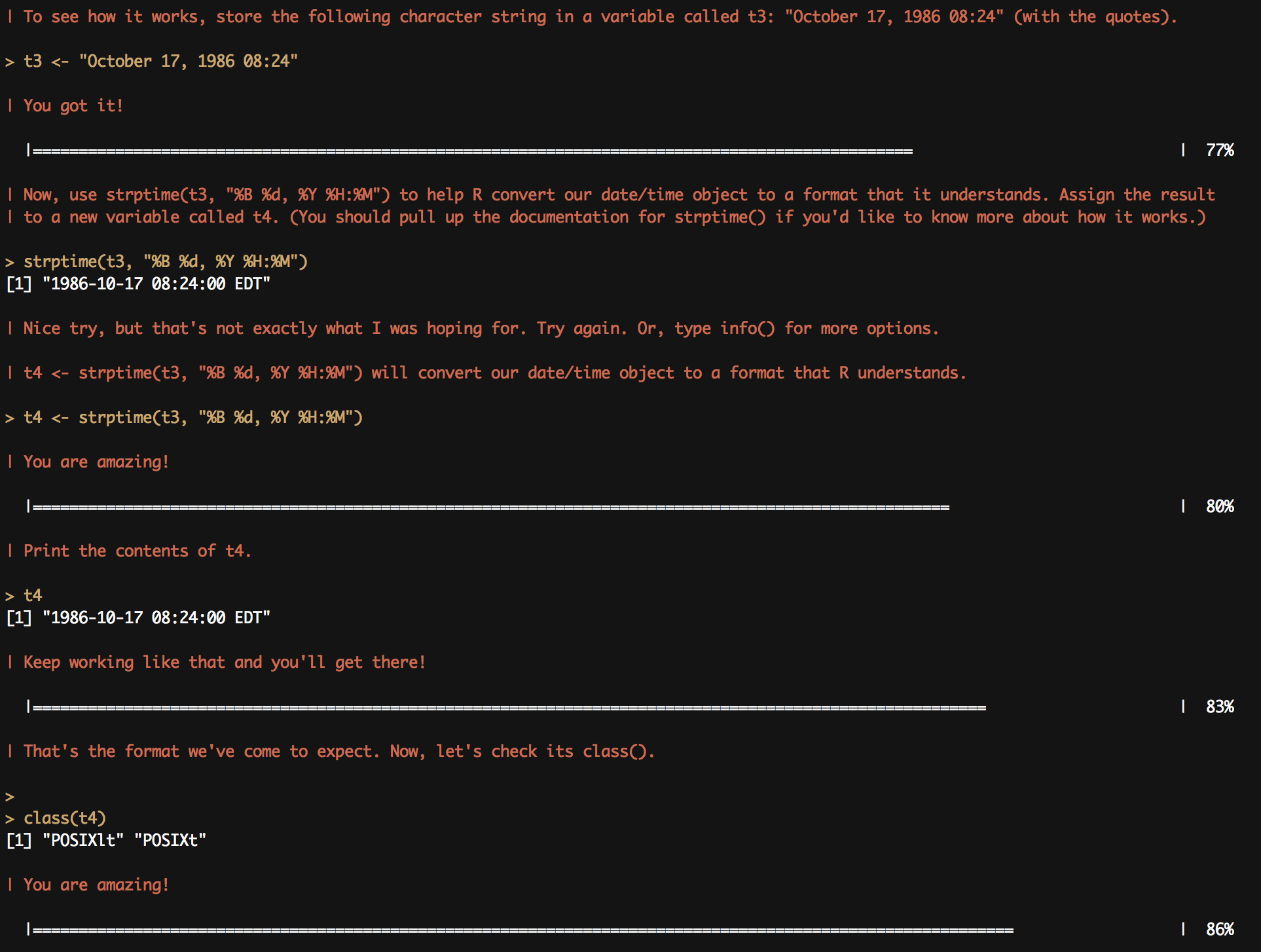# R Hands-On Tutorials with SwirlComing from a Python data analysis background with SciPy, I have always been curious about R.

## Swirl

I decided to check out R and found a handy R package swirl. Swirl "teaches you R programming and data science interactively, at your own pace, and right in the R console".

## Installing Swirl from CRAN

The easiest way to install and run swirl is by typing the following commands in the R console:

```install.packages("swirl")
library(swirl)
swirl()
```

## Lessons

Swirl comes with interactive lessons for the following R topics:

• Basic Building Blocks
• Sequences of Numbers
• Vectors
• Missing Values
• Subsetting Vectors
• Matrices and Data Frames
• Logic
• lapply and sapply
• vapply and tapply
• Looking at Data
• Simulation
• Dates and Times

You can also install the lessons described below from their GitHub repo.

## Beginner Lessons

• R Programming: The basics of programming in R
• Data Analysis: Basic ideas in statistics and data visualization
• Mathematical Biostatistics Boot Camp: One- and two-sample t-tests, power, and sample size
• Open Intro: A very basic introduction to statistics, data analysis, and data visualization

## Intermediate Lessons

• Regression Models: The basics of regression modeling in R
• Getting and Cleaning Data: dplyr, tidyr, lubridate

• Statistical Inference: Introduces the student to basic concepts of statistical inference including probability, hypothesis testing, confidence intervals and p-values. It concludes with an initiation to topics of particular relevance to big data, issues of multiple testing and resampling.

```library(swirl)